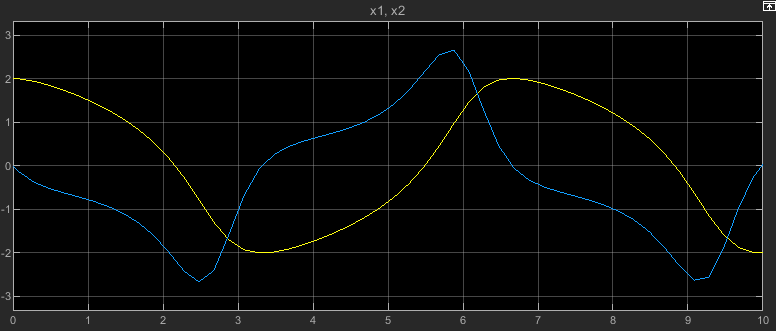# setInitialState

Configure `Simulink.SimulationInput` object to set initial state for simulation

## Syntax

``simIn = setInitialState(simIn,xInitial)``

## Description

example

````simIn = setInitialState(simIn,xInitial)` sets the value of the `InitialState` property for the `Simulink.SimulationInput` object `simIn` using the contents of the variable `xInitial`. Simulations run using the `SimulationInput` object `simIn` start from the initial state or operating point stored in the `InitialState` property of the object.```

## Examples

collapse all

Open the model `vdp`.

```mdl = "vdp"; open_system(mdl);```

Configure the model to save the final operating point at the end of simulation.

1. On the Modeling tab, under Setup, click Model Settings.

2. In the Configuration Parameters dialog box, select the Data Import/Export pane.

3. On the Data Import/Export tab, select Final states and Save final operating point.

4. Click OK.

Alternatively, create a `Simulink.SimulationInput` object to store the parameter values for the simulation. Then, use the `setModelParameter` function to specify the parameter values to use in the simulation.

```simIn = Simulink.SimulationInput(mdl); simIn = setModelParameter(simIn,"SaveFinalState","on"); simIn = setModelParameter(simIn,"SaveOperatingPoint","on");```

Set the stop time for the simulation to 10 seconds. On the Simulation tab, under Simulate, in the Stop Time box, enter `10`, or use the `setModelParameter` function to specify the value of the `StopTime` parameter for the simulation.

`simIn = setModelParameter(simIn,"StopTime","10");`

Simulate the model.

`out = sim(simIn);`

To view the simulation results, double-click the Scope block in the model. The plot in the Scope displays the values of the signals `x1` and `x2` over the 10-second simulation.Resume the simulation by using the operating point you saved at the end of the first simulation as the initial operating point for the second simulation.

Get the final operating point from the first simulation from the `Simulink.SimulationOutput` object `out`.

`vdpOP = out.xFinal;`

Specify the operating point as the initial state for the simulation.

1. On the Modeling tab, under Setup, click Model Settings.

2. In the Configuration Parameters dialog box, on the Data Import/Export pane, select Initial state.

3. In the text box, enter `vdpOP`.

4. Click OK.

Alternatively, create another `Simulink.SimulationInput` object to configure this simulation. Then, use the `setInitialState` function to specify the initial state.

```simIn2 = Simulink.SimulationInput(mdl); simIn2 = setInitialState(simIn2,vdpOP);```

Set the stop time for this simulation to 20. On the Simulation tab, under Simulate, in the Stop Time box, enter `20`, or use the `setModelParameter` function to specify the value of the `StopTime` parameter for the simulation.

`simIn2 = setModelParameter(simIn2,"StopTime","20");`

Simulate the model again, resuming the prior simulation by starting this simulation from the final operating point saved in the first simulation.

`out2 = sim(simIn2);`

The plot in the Scope window updates to show the data from this simulation. The time axis starts at `0` and goes to the simulation stop time of `20`. Because this simulation started from the initial operating point from the first simulation, which ended after 10 simulation seconds, the plot shows the values of the signals `x1` and `x2` only between simulation time 10 seconds and 20 seconds.## Input Arguments

collapse all

Simulation input to specify initial state, specified as a `Simulink.SimulationInput` object.

Initial state or operating point, specified as a `Simulink.op.ModelOperatingPoint` object, a `Simulink.SimulationData.Dataset` object, or a structure that matches the `Structure` or ```Structure with Time``` logging format.

## Output Arguments

collapse all

Simulation configuration with initial states added, returned as a `Simulink.SimulationInput` object.

## Version History

Introduced in R2017a